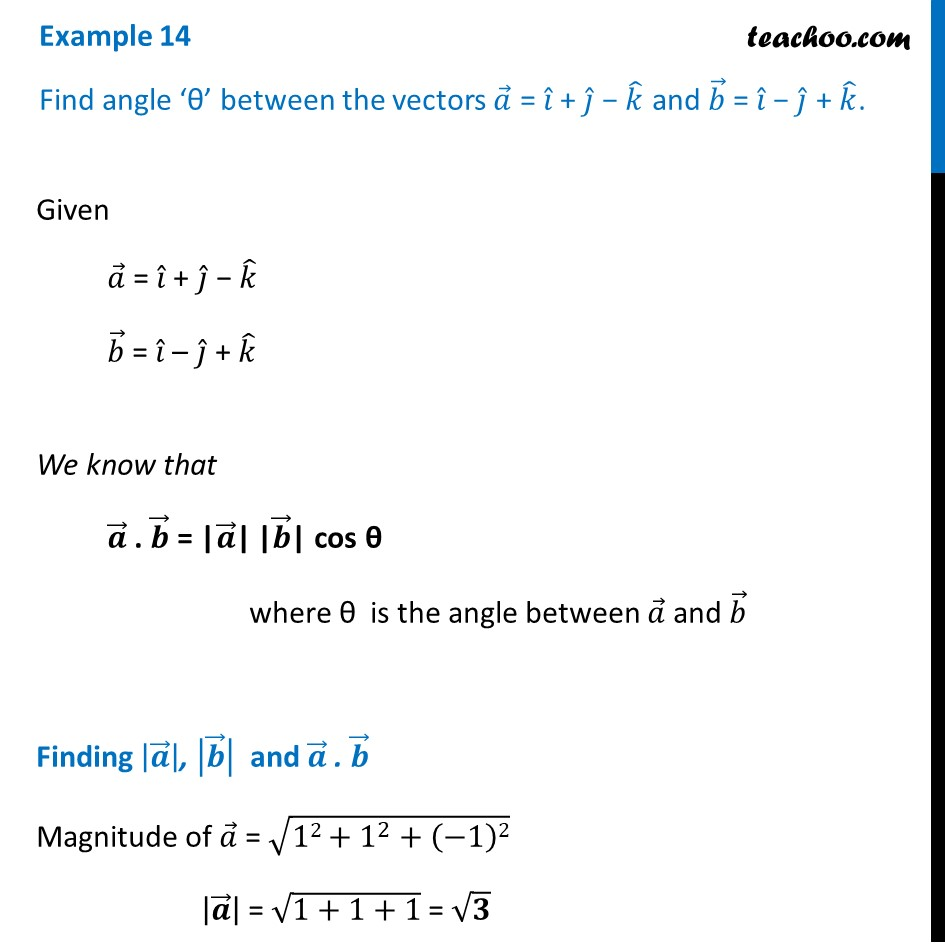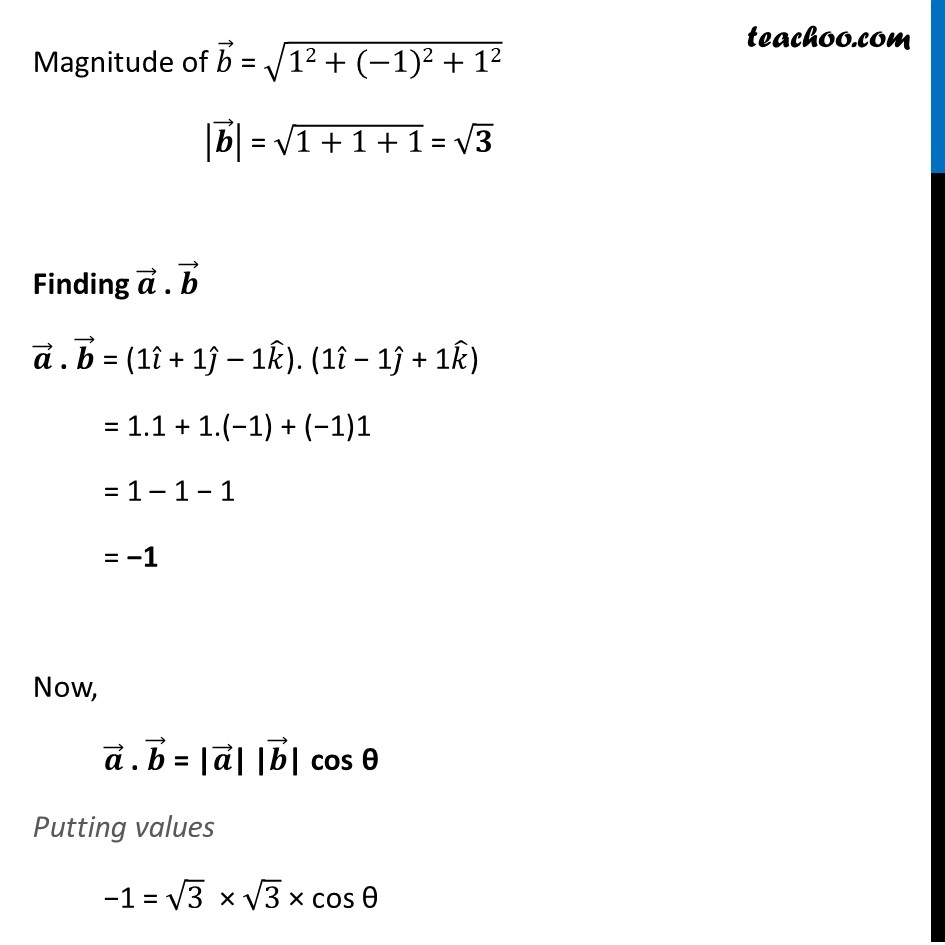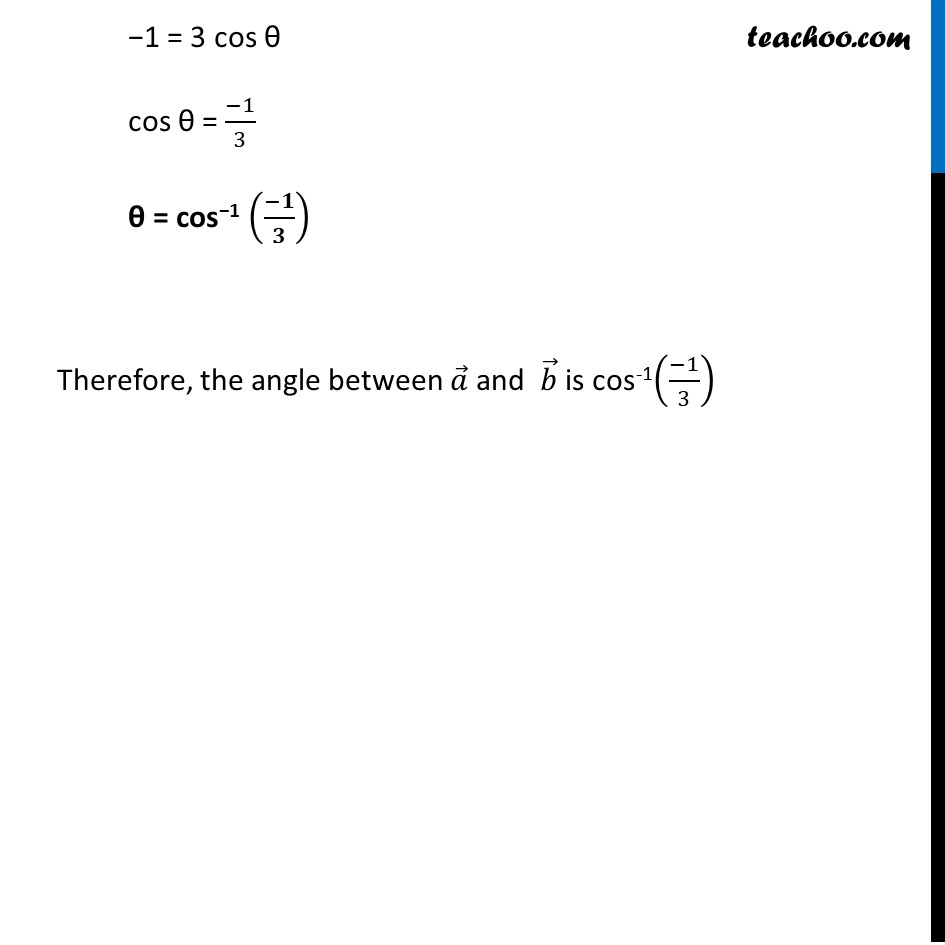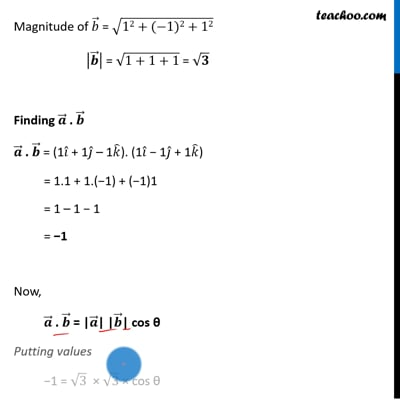Scalar product - Defination

Chapter 10 Class 12 Vector Algebra
Concept wiseThis video is only available for Teachoo black users

Introducing your new favourite teacher - Teachoo Black, at only ₹83 per month

### Transcript

Example 14 Find angle ‘θ’ between the vectors 𝑎 ⃗ = 𝑖 ̂ + 𝑗 ̂ − 𝑘 ̂ and 𝑏 ⃗ = 𝑖 ̂ − 𝑗 ̂ + 𝑘 ̂. Given 𝑎 ⃗ = 𝑖 ̂ + 𝑗 ̂ − 𝑘 ̂ 𝑏 ⃗ = 𝑖 ̂ – 𝑗 ̂ + 𝑘 ̂ We know that 𝒂 ⃗ . 𝒃 ⃗ = "|" 𝒂 ⃗"|" "|" 𝒃 ⃗"|" cos θ where θ is the angle between 𝑎 ⃗ and 𝑏 ⃗ Finding |𝒂 ⃗ |, |𝒃 ⃗ | and 𝒂 ⃗ . 𝒃 ⃗ Magnitude of 𝑎 ⃗ = √(12+1^2+(−1)2) |𝒂 ⃗ | = √(1+1+1) = √𝟑 Magnitude of 𝑏 ⃗ = √(12+(−1)2+12) |𝒃 ⃗ | = √(1+1+1) = √𝟑 Finding 𝒂 ⃗ . 𝒃 ⃗ 𝒂 ⃗ . 𝒃 ⃗ = (1𝑖 ̂ + 1𝑗 ̂ – 1𝑘 ̂). (1𝑖 ̂ − 1𝑗 ̂ + 1𝑘 ̂) = 1.1 + 1.(−1) + (−1)1 = 1 – 1 − 1 = −1 Now, 𝒂 ⃗ . 𝒃 ⃗ = "|" 𝒂 ⃗"|" "|" 𝒃 ⃗"|" cos θ Putting values −1 = √3 × √3 × cos θ −1 = 3 cos θ cos θ = (−1)/3 θ = cos−1 ((−𝟏)/𝟑) Therefore, the angle between 𝑎 ⃗ and 𝑏 ⃗ is cos-1((−1)/3)